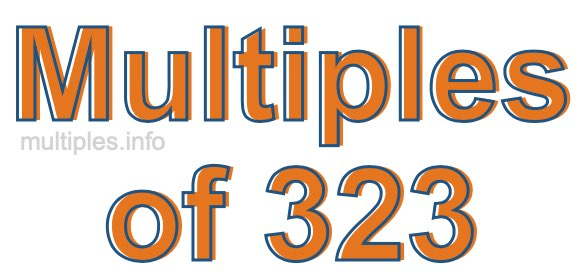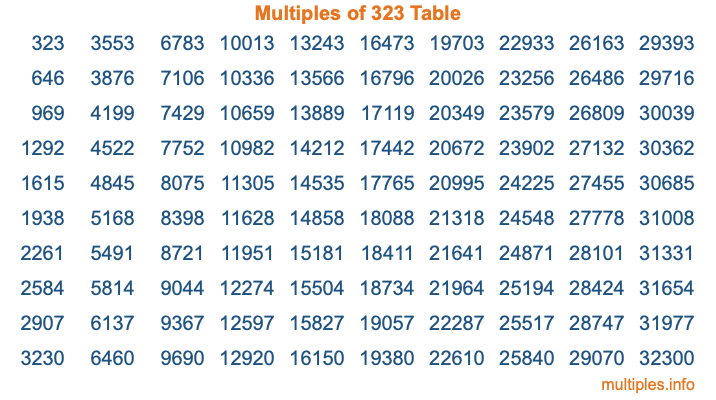Multiples of 323Welcome to the Multiples of 323 page. Here we will first teach you everything you will ever need to know about the multiples of 323, and then give you a study guide summary of everything we taught you to make sure you remember it all. Use this page to look up facts and learn information about the multiples of 323. This page will make you a multiples of three hundred twenty-three expert!

Definition of Multiples of 323
Multiples of 323 are all the numbers that when divided by 323 equal an integer. Each of the multiples of 323 are called a multiple. A multiple of 323 is created by multiplying 323 by an integer.

Therefore, to create a list of multiples of 323, you start with 1 multiplied by 323, then 2 multiplied by 323, then 3 multiplied by 323, and so on for as long as you want. Thus, the list of the first five multiples of 323 is 323, 646, 969, 1292, and 1615. To see a larger list of multiples of 323, see the printable image of Multiples of 323 further down on this page. We also have a category where you can choose any nth multiple of 323.

Multiples of 323 Checker
The Multiples of 323 Checker below checks to see if any number of your choice is a multiple of 323. In other words, it checks to see if there is any number (integer) that when multiplied by 323 will equal your number. To do that, we divide your number by 323. If the the quotient is an integer, then your number is a multiple of 323.

Is  a multiple of 323?

Least Common Multiple of 323 and ...
A Least Common Multiple (LCM) is the lowest multiple that two or more numbers have in common. This is also called the smallest common multiple or lowest common multiple and is useful to know when you are adding our subtracting fractions. Enter one or more numbers below (323 is already entered) to find the LCM.

Check out our LCM Calculator if you need more details about the Least Common Multiple or if you need the LCM for different numbers for adding and subtraction fractions.

nth Multiple of 323
As we stated above, 323 is the first multiple of 323, 646 is the second multiple of 323, 969 is the third multiple of 323, and so on. Enter a number below to find the nth multiple of 323.

th multiple of 323

Multiples of 323 vs Factors of 323
323 is a multiple of 323 and a factor of 323, but that is where the similarities end. All postive multiples of 323 are 323 or greater than 323. All positive factors of 323 are 323 or less than 323.

Below is the beginning list of multiples of 323 and the factors of 323 so you can compare:

Multiples of 323: 323, 646, 969, 1292, 1615, etc.

Factors of 323: 1, 17, 19, 323

As you can see, the multiples of 323 are all the numbers that you can divide by 323 to get a whole number. The factors of 323, on the other hand, are all the whole numbers that you can multiply by another whole number to get 323.

It's also interesting to note that if a number (x) is a factor of 323, then 323 will also be a multiple of that number (x).

Multiples of 323 vs Divisors of 323
The divisors of 323 are all the integers that 323 can be divided by evenly. Below is a list of the divisors of 323.

Divisors of 323: 1, 17, 19, 323

The interesting thing to note here is that if you take any multiple of 323 and divide it by a divisor of 323, you will see that the quotient is an integer.

Multiples of 323 Table
Below is an image of the first 100 multiples of 323 in a table. The table is in chronological order, column by column. The first column has the first ten multiples of 323, the second column has the next ten multiples of 323, and so on.The Multiples of 323 Table is also referred to as the 323 Times Table or Times Table of 323. You are welcome to print out our table for your studies.

Negative Multiples of 323
Although not often discussed or needed in math, it is worth mentioning that you can make a list of negative multiples of 323 by multiplying 323 by -1, then by -2, then by -3, and so on, to get the following list of negative multiples of 323:

-323, -646, -969, -1292, -1615, etc.

Multiples of 323 Summary
Below is a summary of important Multiples of 323 facts that we have discussed on this page. To retain the knowledge on this page, we recommend that you read through the summary and explain to yourself or a study partner why they hold true.

There are an infinite number of multiples of 323.

A multiple of 323 divided by 323 will equal a whole number.

323 divided by a factor of 323 equals a divisor of 323.

The nth multiple of 323 is n times 323.

The largest factor of 323 is equal to the first positive multiple of 323.

323 is a multiple of every factor of 323.

323 is a multiple of 323.

A multiple of 323 divided by a divisor of 323 equals an integer.

323 divided by a divisor of 323 equals a factor of 323.

Any integer times 323 will equal a multiple of 323.

Multiples of a Number
Here you can get the multiples of another number, all with the same attention to detail as we did for multiples of 323 on this page.

Multiples of
Multiples of 324
Did you find our page about multiples of three hundred twenty-three educational? Do you want more knowledge? Check out the multiples of the next number on our list!